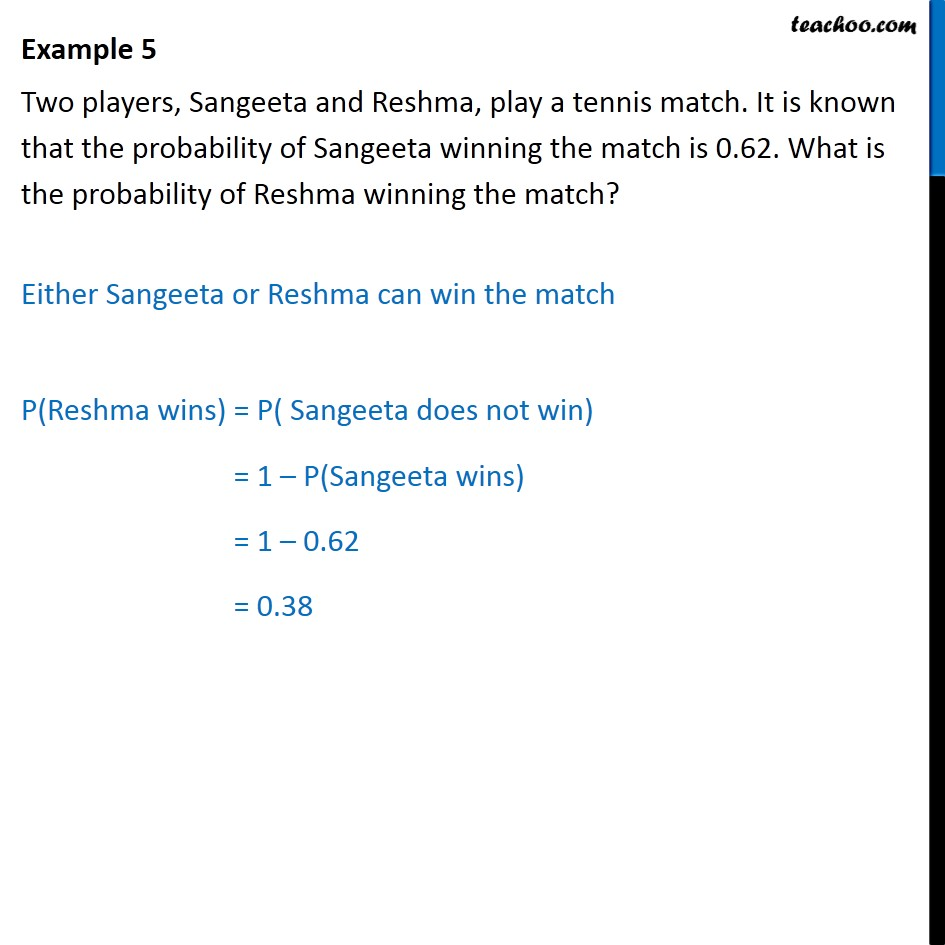1. Chapter 15 Class 10 Probability (Term 1)
2. Serial order wise
3. Examples

Transcript

Example 5 Two players, Sangeeta and Reshma, play a tennis match. It is known that the probability of Sangeeta winning the match is 0.62. What is the probability of Reshma winning the match? Either Sangeeta or Reshma can win the match P(Reshma wins) = P( Sangeeta does not win) = 1 P(Sangeeta wins) = 1 0.62 = 0.38